# Waterfall charts in ggplot2 with waterfalls package

Hugh Parsonage

## Sample data set

Consider the following data set that contains two variables that represent values and its corresponding event, date or group.

``````# install.packages("ggalluvial")
library(ggalluvial)

group <- LETTERS[1:6]
value <- c(2000, 4000, 2000,
-1500, -1000, -2500)
df <- data.frame(x = group, y = value) ``````

## Waterfall graph

In order to create a waterfall chart in ggplot2 making use of the `waterfalls` package you can pass your data frame with groups and values or pass the vectors to the `values` and `labels` arguments of the `waterfall` function.

``````# install.packages("waterfalls")
library(waterfalls)

waterfall(df)

# Equivalent to:
waterfall(values = value, labels = group)``````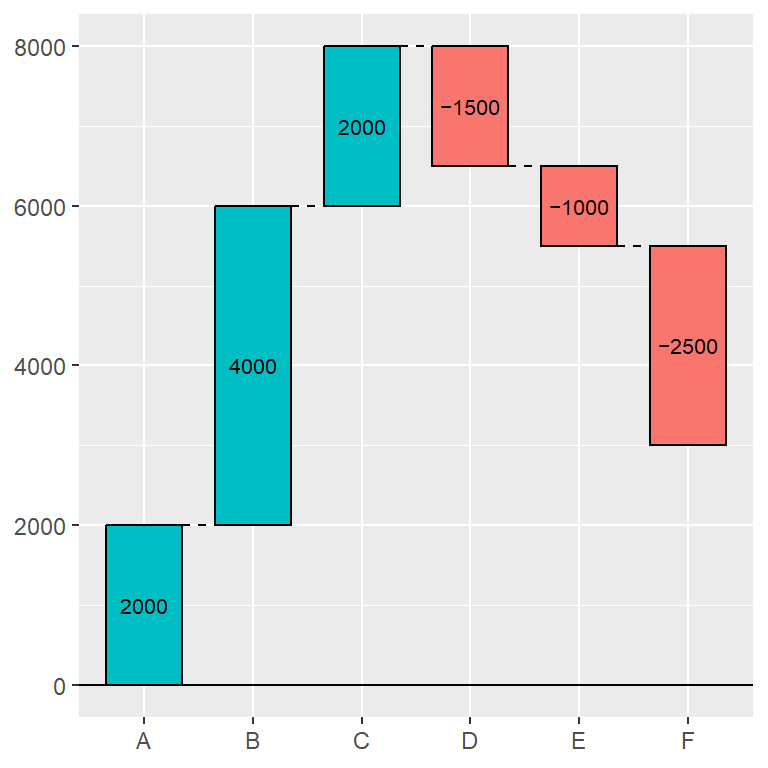Calculate the total

Setting the argument `calc_total` to `TRUE` the final pool will be calculated and a new rectangle will be added to the chart containing the result. You can change the axis name of this rectangle with `total_axis_text`.

``````# install.packages("waterfalls")
library(waterfalls)

waterfall(df, calc_total = TRUE)``````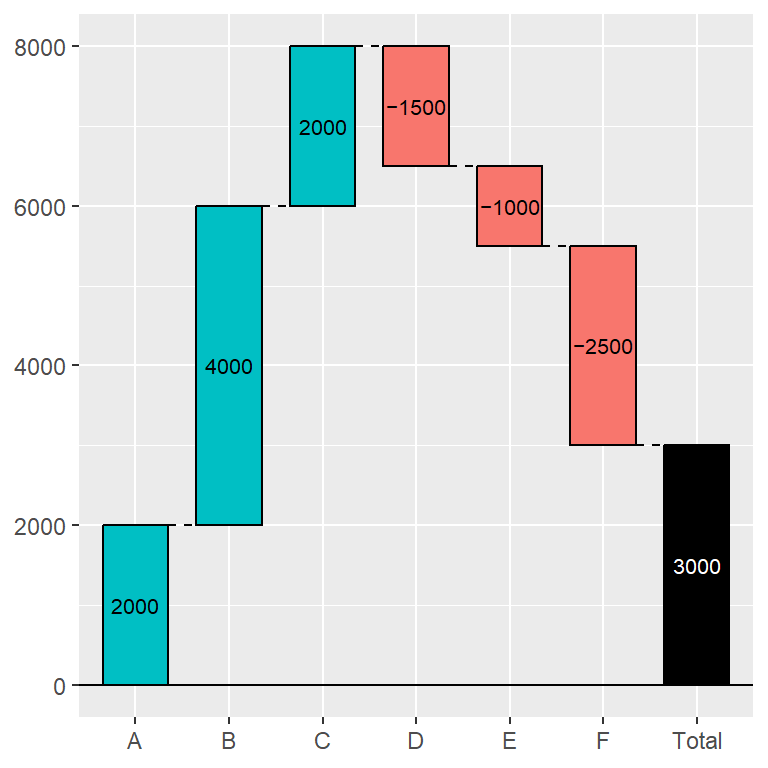Rectangles width

Note that the `rect_width` argument controls the width of the rectangles. The default value is 0.7.

``````# install.packages("waterfalls")
library(waterfalls)

waterfall(df, rect_width = 0.4)``````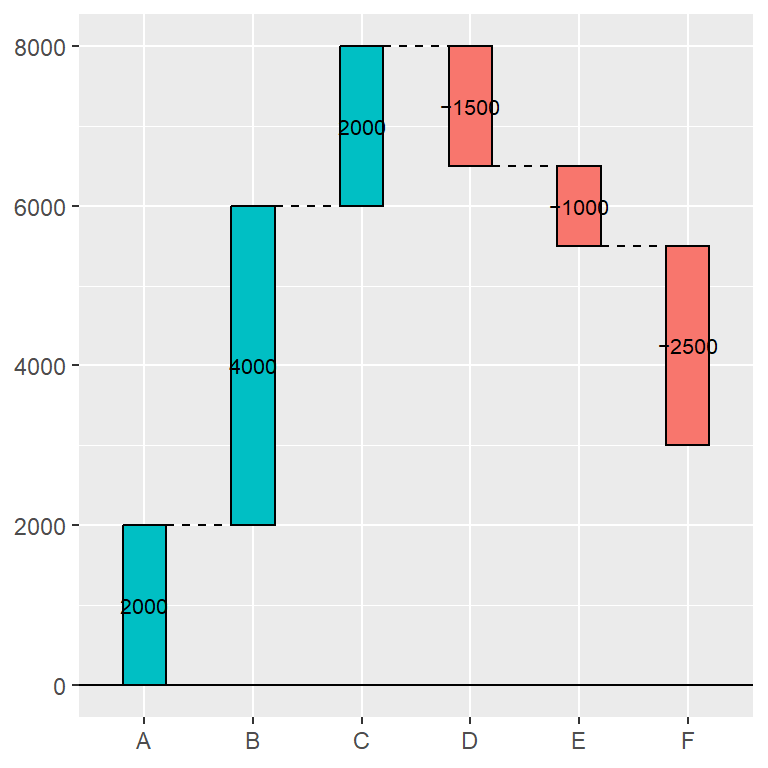Remove lines between rectangles

Between successive rectangles there are dashed lines joining the rectangles. You can remove them setting `draw_lines` to `FALSE`.

``````# install.packages("waterfalls")
library(waterfalls)

waterfall(df, draw_lines = FALSE)``````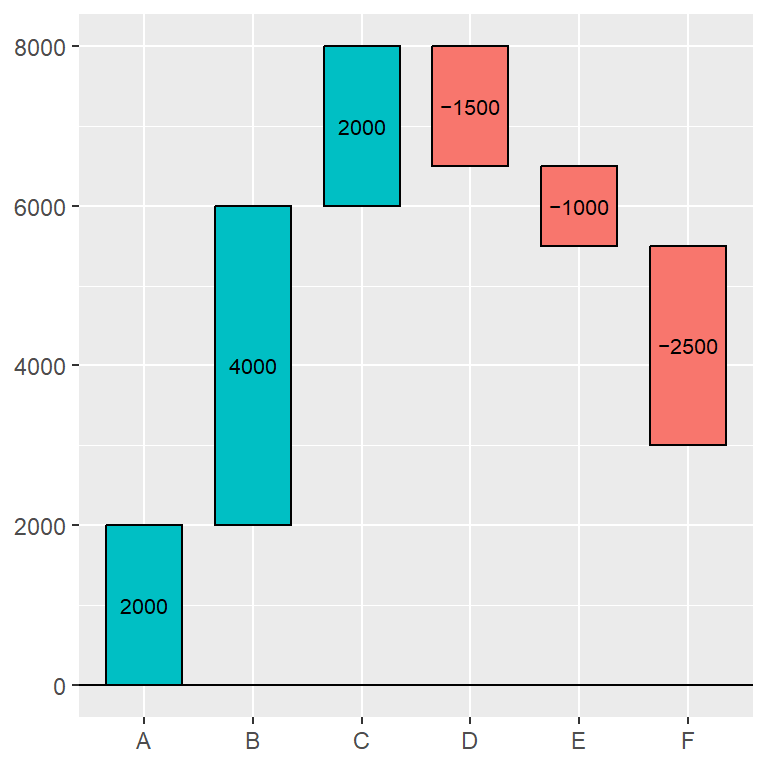Lines type

If you want to keep the lines between the rectangles but prefer to change its line type you can change it with the `linetype` argument.

``````# install.packages("waterfalls")
library(waterfalls)

waterfall(df, linetype = 1)``````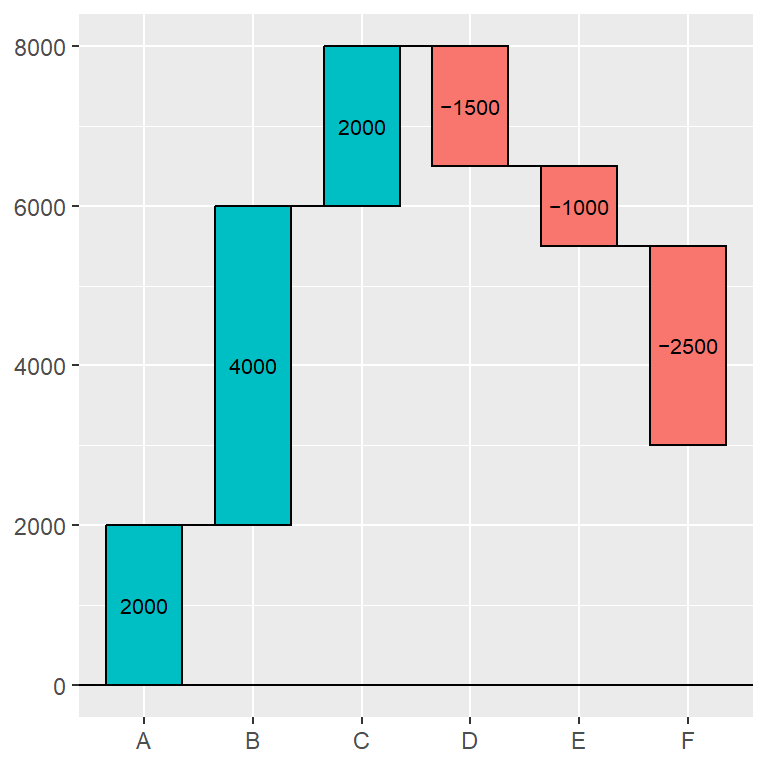## Color customization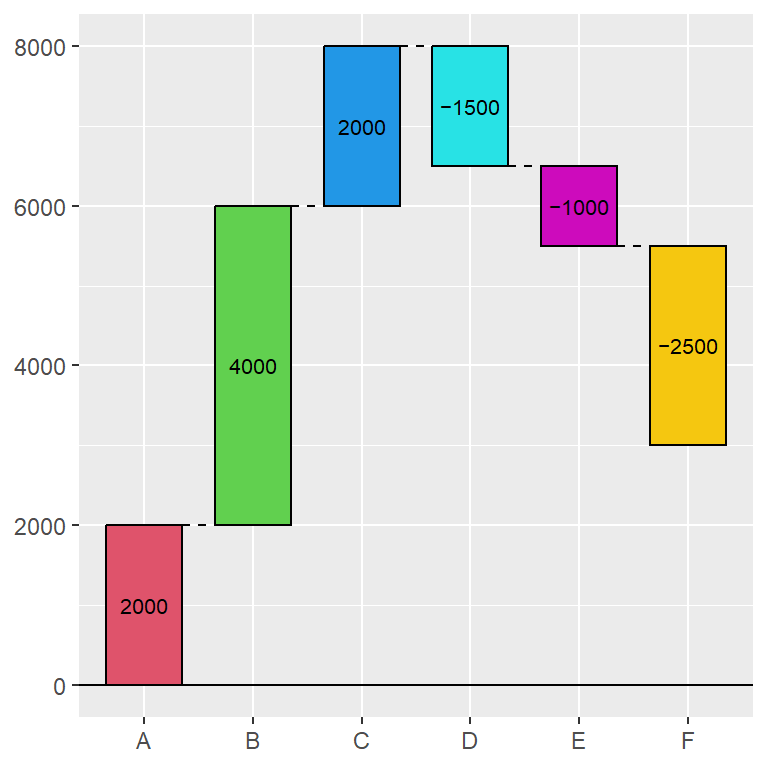Fill the rectangles with custom colors

By default `fill_by_sign = TRUE`, so the positive and negative values will each have the same color. If you set this argument to `FALSE` you can set custom colors with the `fill_colours` argument.

``````# install.packages("waterfalls")
library(waterfalls)

waterfall(df,
fill_by_sign = FALSE,
fill_colours = 2:7)``````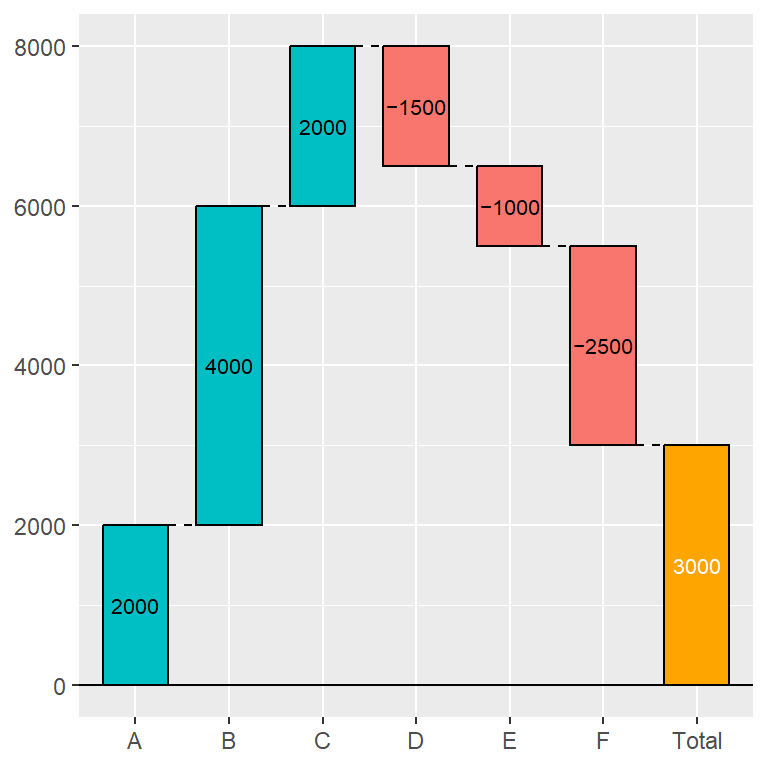Total rectangle colors

If you added the total with `calc_total = TRUE` you can change the color of the rectangle with `total_rect_color` and the color of its text with `total_rect_text_color`.

``````# install.packages("waterfalls")
library(waterfalls)

waterfall(df, calc_total = TRUE,
total_rect_color = "orange",
total_rect_text_color = "white")``````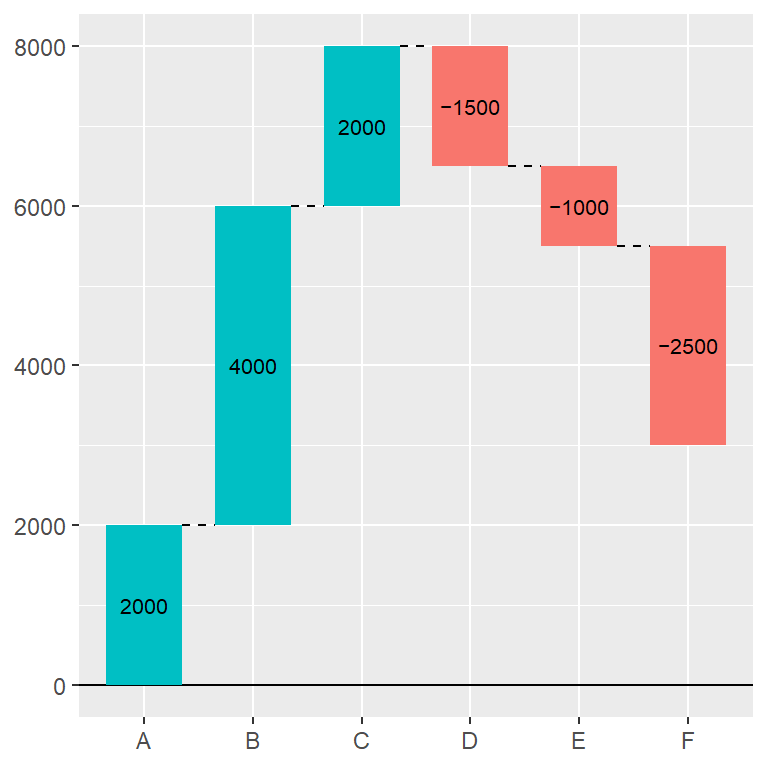Border color

Note that you can also change the color of the border of the rectangles. If you set them to `NA` no borders will be displayed, as shown below.

``````# install.packages("waterfalls")
library(waterfalls)

waterfall(df, rect_border = NA)``````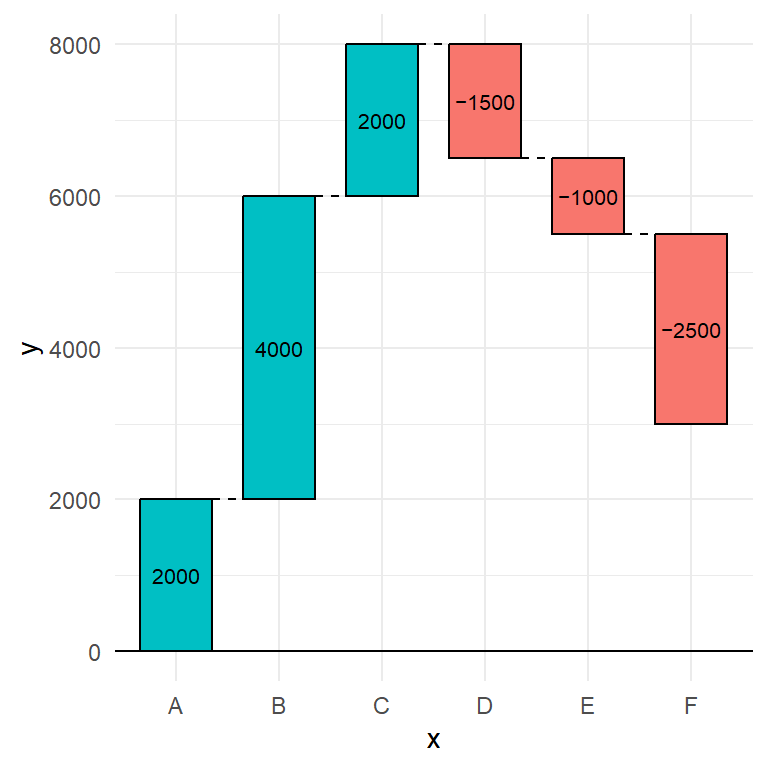Change the theme

Finally, as the package is based on ggplot2 you can add more layers to it, as a title, subtitle or change the default theme, as shown below, among other customizations.

``````# install.packages("waterfalls")
library(waterfalls)
# install.packages("ggplot2)
library(ggplot2)

waterfall(df) +
theme_minimal()``````